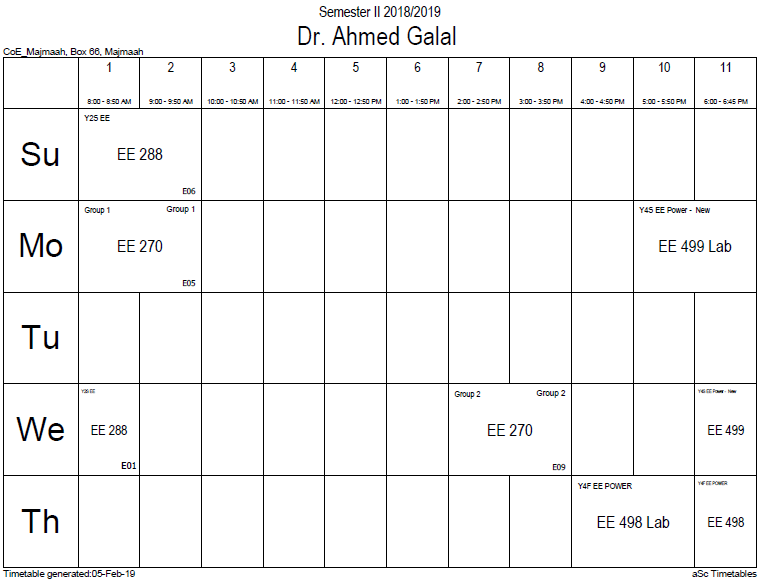## MMF

Similar to the way that EMF drives a current of electrical charge in electrical circuits, magnetomotive force (MMF) 'drives' magnetic flux through magnetic circuits. The term 'magnetomotive force', though, is a misnomer since it is not a force nor is anything moving. It is perhaps better to call it simply MMF. In analogy to the definition of EMF, the magnetomotive force$scriptstyle mathcal{F}$ around a closed loop is defined as:$mathcal{F}=oint mathbf{H} cdot dmathbf{l}.$

The MMF represents the potential that a hypothetical magnetic charge would gain by completing the loop. The magnetic flux that is driven is not a current of magnetic charge; it merely has the same relationship to MMF that electric current has to EMF. (See microscopic origins of reluctance below for a further description.)

The unit of magnetomotive force is the ampere-turn (At), represented by a steady, direct electric current of one ampere flowing in a single-turn loop of electrically conducting material in a vacuum. The gilbert (Gi), established by the IEC in 1930 , is the CGS unit of magnetomotive force and is a slightly smaller unit than the ampere-turn. The unit is named after William Gilbert (1544–1603) English physician and natural philosopher.$egin{matrix}1,operatorname{Gi} & = & {frac {10} {4pi}} mbox{At} \ & approx & 0.795773 mbox{At}end{matrix}$

The magnetomotive force can often be quickly calculated using Ampère's law. For example, the magnetomotive force$mathcal{F}$ of long coil is:$mathcal{F} = N I$,

where N is the number of turns and I is the current in the coil. In practice this equation is used for the MMF of real inductors with N being the winding number of the inducting coil.

An applied MMF 'drives' magnetic flux through the magnetic components of the system. The magnetic flux through a magnetic component is proportional to the number of magnetic field lines that pass through the cross sectional area of that component. This is the net number, i.e. the number passing through in one direction, minus the number passing through in the other direction. The direction of the magnetic field vector B is by definition from the south to the north pole of a magnet inside the magnet; outside the field lines go from north to south.

The flux through an element of area perpendicular to the direction of magnetic field is given by the product of the magnetic field and the area element. More generally, magnetic flux Φ is defined by a scalar product of the magnetic field and the area element vector. Quantitatively, the magnetic flux through a surface S is defined as the integral of the magnetic field over the area of the surface$Phi_m = int !!!! int_S mathbf{B} cdot dmathbf S.$

For a magnetic component the area S used to calculate the magnetic flux Φ is usually chosen to be the cross-sectional area of the component.

The SI unit of magnetic flux is the weber (in derived units: volt-seconds), and the unit of magnetic field is the weber per square meter, or tesla.

### Office HoursNo office hours### My Timetable### Contactsemail: [email protected]

Phone: 2570

### Welcome

Welcome To Faculty of Engineering### IEEEhttp://www.ieee.org/

/

### Bookmarkshttp://www.utk.edu/research/

http://science.doe.gov/grants/index.asp

http://www1.eere.energy.gov/vehiclesandfuels/

http://www.eere.energy.gov/

### Upcoming Conferences### Engineering quotes### Travel Web Siteshttp://www.hotels.com/

http://www.orbitz.com/

http://www.hotwire.com/us/index.jsp

http://www.kayak.com/

### Blackboardستقام اختبارات الميدتيرم يوم الثلاثاء 26-6-1440

حسب الجدول المعلن بلوحات الاعلان

### Summer trainingThe registration for summer training will start from 5th week of second semesterClass registration week 1

### برنامج التجسير### إحصائية الموقع

عدد الصفحات: 2879

البحوث والمحاضرات: 1280

الزيارات: 99544